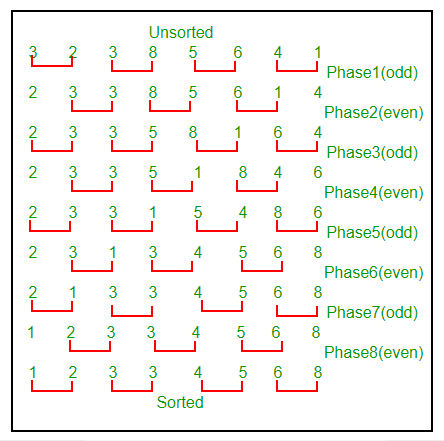Related Articles

# Odd-Even Sort / Brick Sort

• Difficulty Level : Easy
• Last Updated : 28 Jun, 2021

This is basically a variation of bubble-sort. This algorithm is divided into two phases- Odd and Even Phase. The algorithm runs until the array elements are sorted and in each iteration two phases occurs- Odd and Even Phases.
In the odd phase, we perform a bubble sort on odd indexed elements and in the even phase, we perform a bubble sort on even indexed elements.

## C++

 `// A C++ Program to implement Odd-Even / Brick Sort``#include``using` `namespace` `std;` `// A function to sort the algorithm using Odd Even sort``void` `oddEvenSort(``int` `arr[], ``int` `n)``{``    ``bool` `isSorted = ``false``; ``// Initially array is unsorted` `    ``while` `(!isSorted)``    ``{``        ``isSorted = ``true``;` `        ``// Perform Bubble sort on odd indexed element``        ``for` `(``int` `i=1; i<=n-2; i=i+2)``        ``{``            ``if` `(arr[i] > arr[i+1])``             ``{``                ``swap(arr[i], arr[i+1]);``                ``isSorted = ``false``;``              ``}``        ``}` `        ``// Perform Bubble sort on even indexed element``        ``for` `(``int` `i=0; i<=n-2; i=i+2)``        ``{``            ``if` `(arr[i] > arr[i+1])``            ``{``                ``swap(arr[i], arr[i+1]);``                ``isSorted = ``false``;``            ``}``        ``}``    ``}` `    ``return``;``}` `// A utility function ot print an array of size n``void` `printArray(``int` `arr[], ``int` `n)``{``   ``for` `(``int` `i=0; i < n; i++)``       ``cout << arr[i] << ``" "``;``   ``cout << ``"\n"``;``}` `// Driver program to test above functions.``int` `main()``{``    ``int` `arr[] = {34, 2, 10, -9};``    ``int` `n = ``sizeof``(arr)/``sizeof``(arr);` `    ``oddEvenSort(arr, n);``    ``printArray(arr, n);` `    ``return` `(0);``}`

## Java

 `// Java Program to implement``// Odd-Even / Brick Sort``import` `java.io.*;` `class` `GFG``{``    ``public` `static` `void` `oddEvenSort(``int` `arr[], ``int` `n)``    ``{``        ``boolean` `isSorted = ``false``; ``// Initially array is unsorted` `        ``while` `(!isSorted)``        ``{``            ``isSorted = ``true``;``            ``int` `temp =``0``;` `            ``// Perform Bubble sort on odd indexed element``            ``for` `(``int` `i=``1``; i<=n-``2``; i=i+``2``)``            ``{``                ``if` `(arr[i] > arr[i+``1``])``                ``{``                    ``temp = arr[i];``                    ``arr[i] = arr[i+``1``];``                    ``arr[i+``1``] = temp;``                    ``isSorted = ``false``;``                ``}``            ``}` `            ``// Perform Bubble sort on even indexed element``            ``for` `(``int` `i=``0``; i<=n-``2``; i=i+``2``)``            ``{``                ``if` `(arr[i] > arr[i+``1``])``                ``{``                    ``temp = arr[i];``                    ``arr[i] = arr[i+``1``];``                    ``arr[i+``1``] = temp;``                    ``isSorted = ``false``;``                ``}``            ``}``        ``}` `        ``return``;``    ``}``    ``public` `static` `void` `main (String[] args)``    ``{``        ``int` `arr[] = {``34``, ``2``, ``10``, -``9``};``        ``int` `n = arr.length;` `        ``oddEvenSort(arr, n);``        ``for` `(``int` `i=``0``; i < n; i++)``            ``System.out.print(arr[i] + ``" "``);` `        ``System.out.println(``" "``);``    ``}``}``// Code Contribute by Mohit Gupta_OMG <(0_o)>`

## Python3

 `# Python Program to implement``# Odd-Even / Brick Sort` `def` `oddEvenSort(arr, n):``    ``# Initially array is unsorted``    ``isSorted ``=` `0``    ``while` `isSorted ``=``=` `0``:``        ``isSorted ``=` `1``        ``temp ``=` `0``        ``for` `i ``in` `range``(``1``, n``-``1``, ``2``):``            ``if` `arr[i] > arr[i``+``1``]:``                ``arr[i], arr[i``+``1``] ``=` `arr[i``+``1``], arr[i]``                ``isSorted ``=` `0``                ` `        ``for` `i ``in` `range``(``0``, n``-``1``, ``2``):``            ``if` `arr[i] > arr[i``+``1``]:``                ``arr[i], arr[i``+``1``] ``=` `arr[i``+``1``], arr[i]``                ``isSorted ``=` `0``    ` `    ``return`  `arr ``=` `[``34``, ``2``, ``10``, ``-``9``]``n ``=` `len``(arr)` `oddEvenSort(arr, n);``for` `i ``in` `range``(``0``, n):``    ``print``(arr[i], end ``=` `' '``)``    ` `# Code Contribute by Mohit Gupta_OMG <(0_o)>`

## C#

 `// C# Program to implement``// Odd-Even / Brick Sort``using` `System;` `class` `GFG``{``    ``public` `static` `void` `oddEvenSort(``int` `[]arr, ``int` `n)``    ``{``        ``// Initially array is unsorted``        ``bool` `isSorted = ``false``;` `        ``while` `(!isSorted)``        ``{``            ``isSorted = ``true``;``            ``int` `temp =0;` `            ``// Perform Bubble sort on``            ``// odd indexed element``            ``for` `(``int` `i = 1; i <= n - 2; i = i + 2)``            ``{``                ``if` `(arr[i] > arr[i+1])``                ``{``                    ``temp = arr[i];``                    ``arr[i] = arr[i+1];``                    ``arr[i+1] = temp;``                    ``isSorted = ``false``;``                ``}``            ``}` `            ``// Perform Bubble sort on``            ``// even indexed element``            ``for` `(``int` `i = 0; i <= n - 2; i = i + 2)``            ``{``                ``if` `(arr[i] > arr[i+1])``                ``{``                    ``temp = arr[i];``                    ``arr[i] = arr[i+1];``                    ``arr[i+1] = temp;``                    ``isSorted = ``false``;``                ``}``            ``}``        ``}``        ``return``;``    ``}``    ` `    ``// Driver code``    ``public` `static` `void` `Main ()``    ``{``        ``int` `[]arr = {34, 2, 10, -9};``        ``int` `n = arr.Length;``        ` `        ``// Function calling``        ``oddEvenSort(arr, n);``        ``for` `(``int` `i = 0; i < n; i++)``            ``Console.Write(arr[i] + ``" "``);` `        ``Console.WriteLine(``" "``);``    ``}``}` `// This code is contributed by Sam007`

## Javascript

 ``

Output :

`-9 2 10 34 `

We demonstrate the above algorithm using the below illustration on the array = {3, 2, 3, 8, 5, 6, 4, 1}Please refer wiki for proof of correctness.
Time Complexity : O(N2) where, N = Number of elements in the input array.
Auxiliary Space : O(1). Just like bubble sort this is also an in-place algorithm.
Exercise
In our program in each iteration we first do bubble sort on odd indexed elements and then a bubble sort on the even indexed elements.
Will we get a sorted result if we first perform a bubble sort on even indexed element first and then on the odd indexed element ?
References
https://en.wikipedia.org/wiki/Odd%E2%80%93even_sort
This article is contributed by Rachit Belwariar. If you like GeeksforGeeks and would like to contribute, you can also write an article and mail your article to review-team@geeksforgeeks.org. See your article appearing on the GeeksforGeeks main page and help other Geeks.